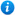# Year 1 Addition and Subtraction Bundle

Year 1 Addition and Subtraction Bundle

Year 1 maths programme of study - Number (addition and subtraction)

Pupils are taught to:

• read, write and interpret mathematical statements involving addition (+), subtraction (-) and equals (=) signs
• add and subtract one-digit and two-digit numbers to 20, including 0
• solve one-step problems that involve addition and subtraction, using concrete objects and pictorial representations

This year 1 maths bundle introduces pupils to addition and subtraction as per the curriculum objectives of the year 1 maths programme of study listed above. It contains 8 PowerPoint presentations with accompanying worksheets. These include:

2. Adding Using a Number Line
3. Addition and Subtraction are Opposites!
5. Let’s Take Away - Beginning to Subtract
6. Number Fact Families
7. Taking Away Using a Number Line
8. Up, Up and Take Away! (Subtracting Numbers to 20)

This bundle gives you a saving of 52% when compared to buying each resource individually.

For a full description of each resource please click on the image of the included resources below.

Our Price : £8.99 / 10 Credits## Resources included (8)

£2.00
32 powerpoint slides | 3 worksheets
£0.00
28 powerpoint slides
£1.99
Addition and Subtraction are Opposites - Year 1
34 powerpoint slides | 2 worksheets
£2.99
Addition and Subtraction Facts - Year 1
42 powerpoint slides | 5 worksheets
£2.99
Number Fact Families
36 powerpoint slides | 4 worksheets
£1.50
Taking Away Using a Number Line - Year 1
18 powerpoint slides | 2 worksheets
£1.99
Up, Up and Take Away
35 powerpoint slides
£2.99
Beginning to Subtract
41 powerpoint slides | 5 worksheets

By continuing to use the site, you agree to the use of cookies. You can change this and find out more by following this link